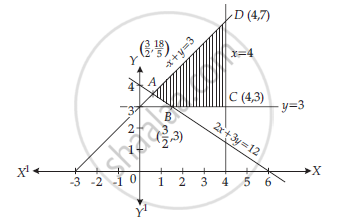Advertisement Remove all ads

# Solve the Following Using Graphical Method : Minimize :Z=3x+5y 2 X + 3 X ≥ 12 − X + Y ≤ 3 X ≤ 4 , Y ≥ 3 , X ≥ 0 , Y ≥ 0 - Mathematics and Statistics

Solve the following using graphical method :

Minimize :Z=3x+5y

2x+3x>=12

-x+y<=3

x<=4,y>=3,x>=0,y>=0

Advertisement Remove all ads

#### Solution

Min Z = 3x + 5y
S.t. 2x + 3y ≥ 12                    …(i)
– x + y ≤ 3                             …(ii)
x ≤ 4, y ≥ 3, x ≥ 0, y ≥ 0

Taking eqn (i)
2x + 3y = 12
Putting x = 0, y = 4 Let the point is (0, 4)
Now putting y = 0, x = 6 Let the point is (6, 0)
Now taking eqn (ii)
– x + y = 3
Putting x = 0, y = 3 (0, 3)
Putting y = 0, x = – 3 (– 3, 0)
The graph is as followsABCDA be the feasible region bounded by these
lines Now we find the coordinates of A, B, C and D
for A, Solving the eqns.

2x+3y=12 and -xy=3

We get

x=(+3)/5  and  y=18/5

coordinate of A((+3)/5,18/5)

Now

Z=3xx(+3/5)+5xx18/5

=(+9)/5+90/5=90/5

For B, Solving the eqns

2x+3y=12 and y=3

We get x=3/2,y=3

∴Coordinate of B (3/2,3)

Now Z=3xx3/2+5xx3

=9/2+15=39/2

For C. Solving the eqn x = 4 and y = 3

∴ Coordinate of C (4, 3)

Now Z=3xx4+5xx3

=12+1=27

For D, Solving the eqn

– x + y = 3 and x = 4
We get  x = 4, y = 7

Now Z = 3 × 4 + 5 × 7
= 12 + 35 = 47

Min Z = 39/2, "for" x=3/2,y=3

Concept: Linear Programming Problem in Management Mathematics
Is there an error in this question or solution?
Advertisement Remove all ads

#### APPEARS IN

Advertisement Remove all ads

#### Video TutorialsVIEW ALL 

Advertisement Remove all ads
Share
Notifications

View all notifications

Forgot password?